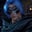Related Tags

java
hashmap
anagram
communitycreator

# How to check if two strings are anagram using HashMap in JavaGutha Vamsi Krishna

### Problem

Check whether the given two strings are an anagram of each other using HashMap in Java.

From Wikipedia:

An anagram is a phrase or word formed by rearranging letters, usually using the original letters exactly once. For example, the word anagram itself can be rearranged into nag a ram, also the word binary into brainy, and the word adobe into the abode.

### Example

Input: race, care

Output: The two strings are anagrams of each other

Explanation: By rearranging race, we can get care, so they are anagrams of each other.

Input: down, drown

Output: The two strings are NOT anagrams of each other

Explanation: By rearranging down, we cannot get drown, so they are NOT anagrams.

### Solution

• Take the first hashmap and fill the first string's individual characters as key and value as the character count in the string.
• Take the second hashmap, and fill the second string's individual characters as key and value as the character count in the string.
• Check if two hashmaps are equal or not. If they are equal, then the strings are anagrams of each other. Otherwise, they are not.

### Code

import java.io.*;
import java.util.*;

class Solution {
public static void main(String args[]) {

String str1 = "race";
String str2 = "care";
// initialize hashmaps
HashMap < Character, Integer > hashmap1 = new HashMap < Character, Integer > ();
HashMap < Character, Integer > hashmap2 = new HashMap < Character, Integer > ();
//convert string to character array
char arr1[] = str1.toCharArray();
char arr2[] = str2.toCharArray();
//for loop for first string
for (int i = 0; i < arr1.length; i++) {
//if character not present add to hashmap
if (hashmap1.get(arr1[i]) == null) {

hashmap1.put(arr1[i], 1);
} else {
Integer c = (int) hashmap1.get(arr1[i]);
hashmap1.put(arr1[i], ++c);
}
}
//for loop for second string
for (int j = 0; j < arr2.length; j++) {

if (hashmap2.get(arr2[j]) == null)
hashmap2.put(arr2[j], 1);
else {

Integer d = (int) hashmap2.get(arr2[j]);
hashmap2.put(arr2[j], ++d);
}
}
//check if hashmaps are equal
if (hashmap1.equals(hashmap2))
System.out.println("The two strings are anagrams of each other");
else
System.out.println("The two strings are NOT anagrams of each other");

}
}

### Explanation

• Line 7 and 8: Initialize two strings, str1 and str2, with race and care, respectively.

• Line 10 and 11: Initialize two hashmaps, hasmap1 and hashmap2, which has the key of the Character type, and value of the Integer type.

• Line 13 and 14: Convert strings to the character array and store them in character array variables, arr1 and arr2.

• Lines 16 to 25 and 27 to 36: Use two for loops to traverse both character arrays individually,

• While traversing, check if the present character is already present in the hashmap. If it isn’t, add it to hashmap. If it is, increase the count of that character in the hashmap.
• After both the loops have been completely executed, check if two hashmaps are equal or not. If they are equal, print The two strings are anagrams of each other. Otherwise, The two strings are NOT anagrams of each other.

RELATED TAGS

java
hashmap
anagram
communitycreator

CONTRIBUTORGutha Vamsi Krishna
RELATED COURSES

View all Courses

Keep Exploring

Learn in-demand tech skills in half the time